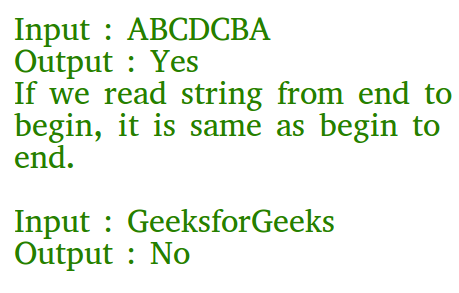# C Program to Check if a Given String is Palindrome

• Difficulty Level : Easy
• Last Updated : 23 Jun, 2022

Given a string, write a c function to check if it is palindrome or not.

A string is said to be palindrome if reverse of the string is same as string. For example, “abba” is palindrome, but “abbc” is not palindrome.Recommended Practice

Algorithm:

isPalindrome(str)

1. Find length of str. Let length be n.
2. Initialize low and high indexes as 0 and n-1 respectively.
3.  Do following while low index ‘l’ is smaller than high index ‘h’.
• If str[l] is not same as str[h], then return false.
• Increment l and decrement h, i.e., do l++ and h–.
4. If we reach here, it means we didn’t find a mis

Following is C implementation to check if a given string is palindrome or not.

## C

 `#include ` `#include `   `// A function to check if a string str is palindrome` `void` `isPalindrome(``char` `str[])` `{` `    ``// Start from leftmost and rightmost corners of str` `    ``int` `l = 0;` `    ``int` `h = ``strlen``(str) - 1;`   `    ``// Keep comparing characters while they are same` `    ``while` `(h > l)` `    ``{` `        ``if` `(str[l++] != str[h--])` `        ``{` `            ``printf``(``"%s is not a palindrome\n"``, str);` `            ``return``;` `        ``}` `    ``}` `    ``printf``(``"%s is a palindrome\n"``, str);` `}`   `// Driver program to test above function` `int` `main()` `{` `    ``isPalindrome(``"abba"``);` `    ``isPalindrome(``"abbccbba"``);` `    ``isPalindrome(``"geeks"``);` `    ``return` `0;` `}`

Output

```abba is a palindrome
abbccbba is a palindrome
geeks is not a palindrome```

Time complexity : O(n)
Auxiliary Space : O(1)

Recursive function to check if a string is palindrome

My Personal Notes arrow_drop_up
Recommended Articles
Page :